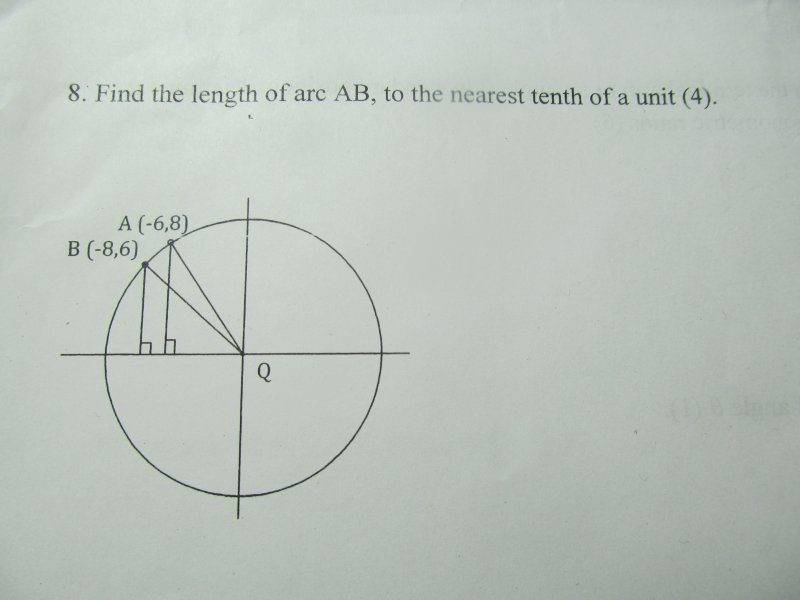# Trigonometry Arc Length Problem

First I'd just like to point out that I'm taking calculus and advance pre-calculus simultaneously (kind of a stupid system) and this is a problem in the pre-calc.

1. Homework Statement2. Homework Equations

Let 'a' be arc length.

$$a=\theta r$$

$$a = \int_{a}^{b} \sqrt{1+[f'(x)]^{2}} dx$$

3. The Attempt at a Solution

The lecture on arc length wasn't even a lecture. Merely a hand out of the chapter summery, not to be touched again. I saw no central angle so $$a=\theta r$$ seems to be out of the question. This seemed to be an unusual problem, so I used Pythagoras after a while. I went

$$( \Delta x )^{2} + (\Delta y)^{2} = a^{2}$$

This yields the arc length to $$\sqrt{8}$$ units.

I confirmed this with the integration for arc length. But I'm really not at the level of arc length integration yet. I can do basic integration only (I skipped ahead in the course a bit). Via wolfram alpha:

$$\int_{-8}^{-6} 10\sqrt{\frac{1}{100-x^{2}}} dx = 10\sin ^{-1}\left(\frac{7}{25}\right) \approx 2.83794$$

Which is pretty much $$\sqrt{8}$$

So really, my question is if there is another more formal way to approach this problem using trigonometric concepts to arrive at an answer.

phinds
Gold Member
2021 Award
Can you figure out the angle AQB?

•Euler2718
Can you figure out the angle AQB?

Ahhh. Using the right triangles no doubt. I'll give it a go. Thanks.

Mark44
Mentor
Problems involving derivatives or integrals should be posted in the calculus section, not in the precalculus section. I have moved this thread.

Problems involving derivatives or integrals should be posted in the calculus section, not in the precalculus section. I have moved this thread.

Sorry. I merely included the calculus to show what I used to compute the answer, as it was technically part of my attempt.

Pretty sure I got it now! Thank you phinds for the tip. I calculated the central angle to be 16.3°, and used the arc length equation from there. It comes out to 2.8 units, which is what the Pythagorean approximation and the integration came to.

phinds
•